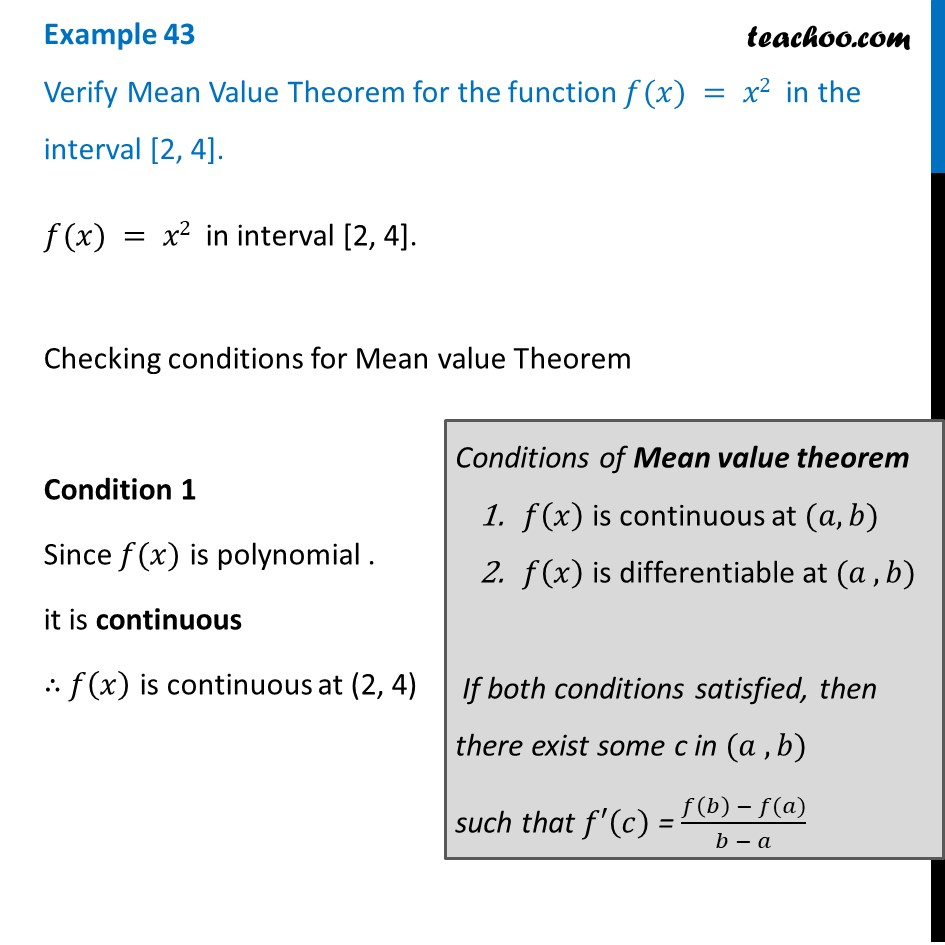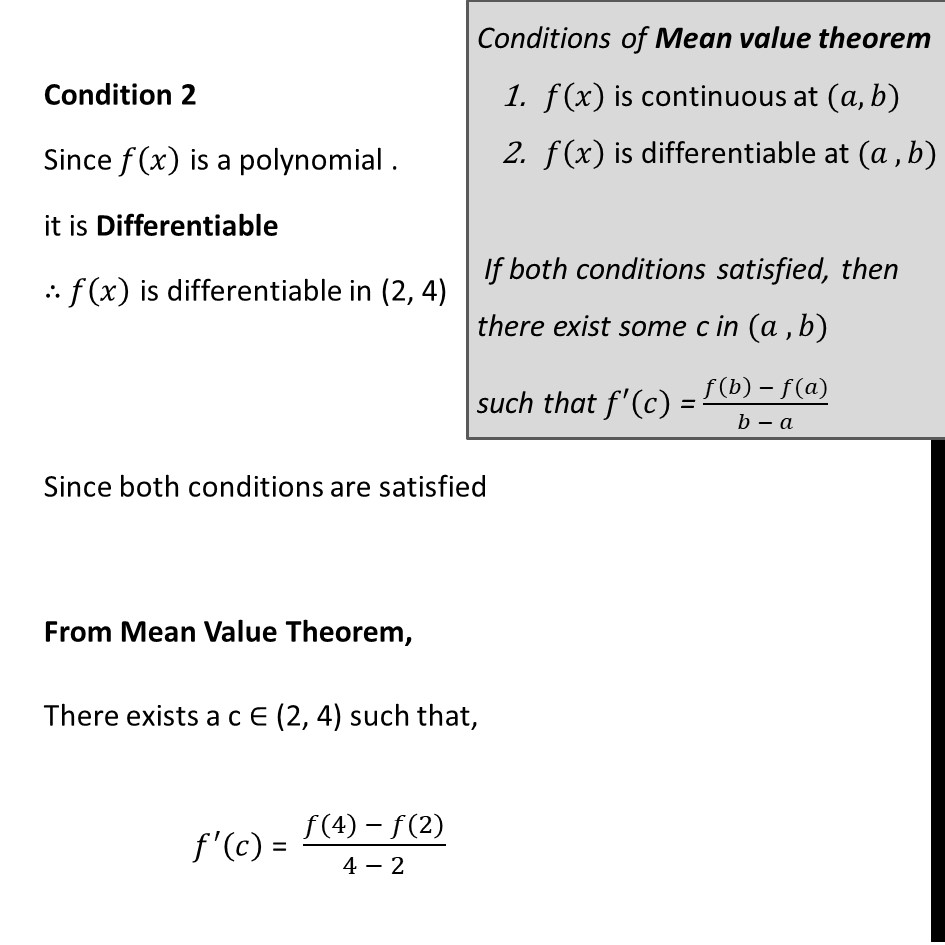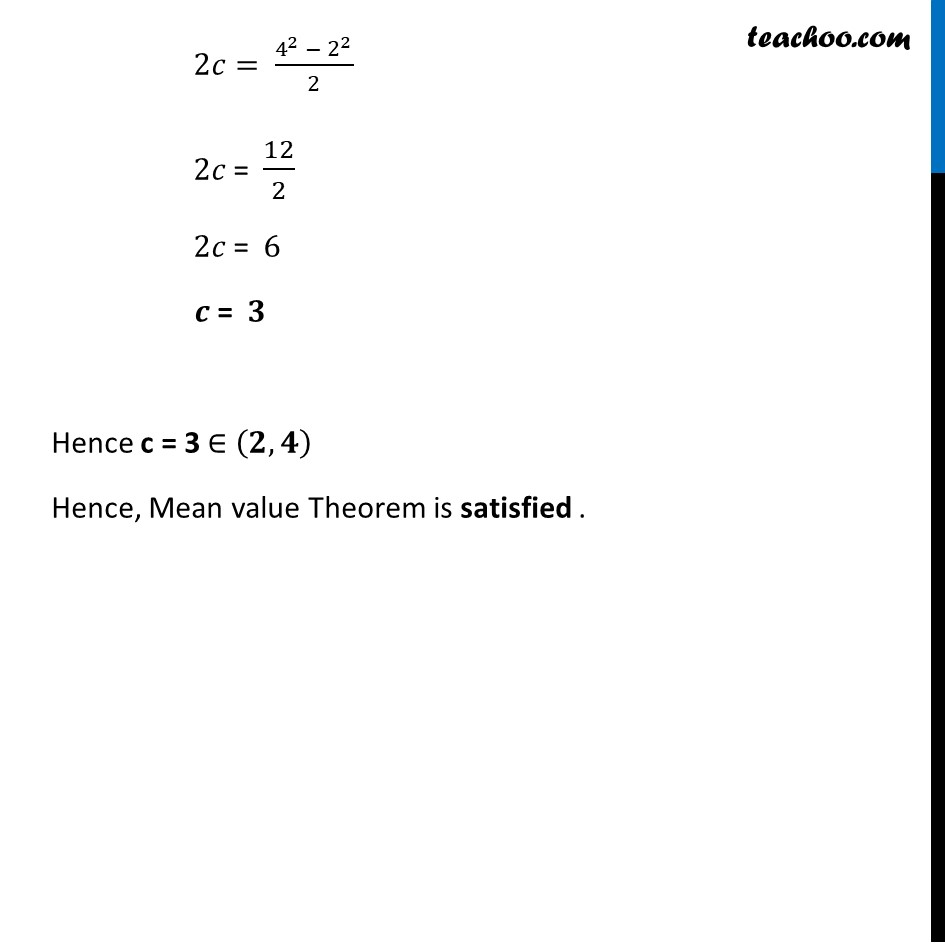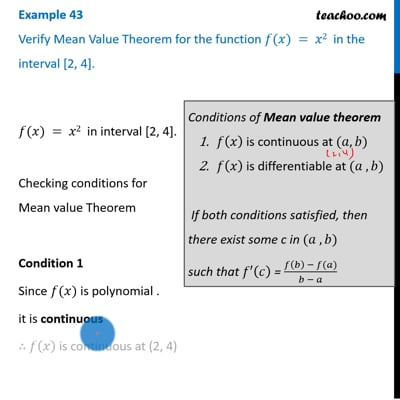Verify Mean Value Theorem

Chapter 5 Class 12 Continuity and Differentiability
Concept wiseThis video is only available for Teachoo black users

Learn in your speed, with individual attention - Teachoo Maths 1-on-1 Class

### Transcript

Question 5 Verify Mean Value Theorem for the function 𝑓(𝑥) = 𝑥2 in the interval [2, 4]. 𝑓(𝑥) = 𝑥2 in interval [2, 4]. Checking conditions for Mean value Theorem Condition 1 Since 𝑓(𝑥) is polynomial . it is continuous ∴ 𝑓(𝑥) is continuous at (2, 4) Conditions of Mean value theorem 𝑓(𝑥) is continuous at (𝑎, 𝑏) 𝑓(𝑥) is differentiable at (𝑎 , 𝑏) If both conditions satisfied, then there exist some c in (𝑎 , 𝑏) such that 𝑓′(𝑐) = (𝑓(𝑏) − 𝑓(𝑎))/(𝑏 − 𝑎)Condition 2 Since 𝑓(𝑥) is a polynomial . it is Differentiable ∴ 𝑓(𝑥) is differentiable in (2, 4) Since both conditions are satisfied From Mean Value Theorem, There exists a c ∈ (2, 4) such that, 𝑓^′ (𝑐) = (𝑓(4) − 𝑓(2))/(4 − 2) Conditions of Mean value theorem 𝑓(𝑥) is continuous at (𝑎, 𝑏) 𝑓(𝑥) is differentiable at (𝑎 , 𝑏) If both conditions satisfied, then there exist some c in (𝑎 , 𝑏) such that 𝑓′(𝑐) = (𝑓(𝑏) − 𝑓(𝑎))/(𝑏 − 𝑎) Condition 2 Since 𝑓(𝑥) is a polynomial . it is Differentiable ∴ 𝑓(𝑥) is differentiable in (2, 4) Since both conditions are satisfied From Mean Value Theorem, There exists a c ∈ (2, 4) such that, 𝑓^′ (𝑐) = (𝑓(4) − 𝑓(2))/(4 − 2) 2𝑐= (4^2 − 2^2)/2 2𝑐 = 12/2 2𝑐 = 6 𝒄 = 𝟑 Hence c = 3 ∈(𝟐, 𝟒) Hence, Mean value Theorem is satisfied .